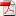| English | Deutsch

# HEXAGON Newsletter 178 - Nov./Dec. 2019

#### by Fritz Ruoss

WN5 – Tolerance fit H / js and H / k added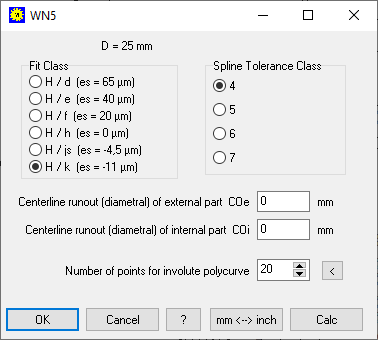The ISO tolerances js and k for external involute splines have been added under Edit \ Quality. As fit with the internal toothed spline (ISO tolerance zone H) this results in transition fits.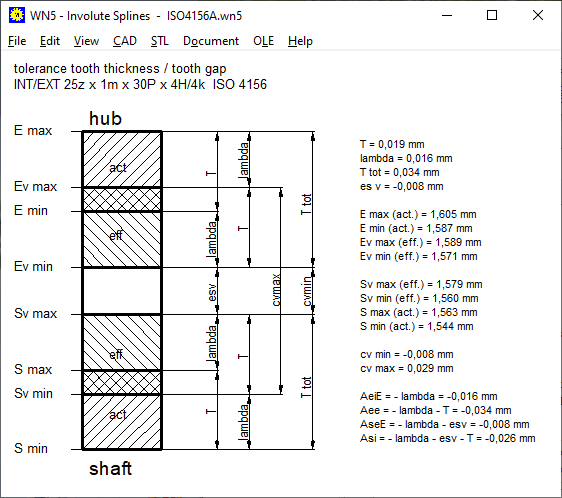This means that the backlash c can be positive or negative. Depending on the tolerances, the fit has backlash or pressure.

WL1 +: X-O arrangement also for cylindrical roller bearingsNJ cylindrical roller bearings are axially held on only one side. For this type of cylindrical roller bearing you have to choose "radial thrust bearing" and specify X or O arrangement, that the bearing is shown in the drawing in the correct direction. Depending on the direction of the axial force, this is either absorbed by the right or left bearing.

WL1 +: STL layer model for 3D printing

If the shaft is printed without a hole in 3D printing of hollow shafts, you can now alternatively print the shaft in layers under "STL \ Shaft (sliced)". The layer thickness can be configured under Settings \ CAD \ zslice. If the stl file size or loading time is too large, you can increase the values for layer thickness (zslice) and circular arc accuracy (arc-> lines precision).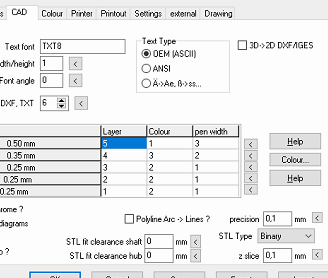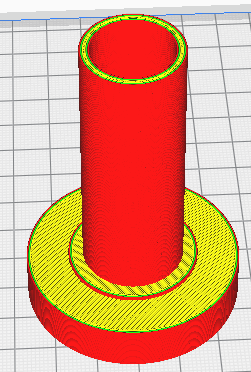GR2: Interfaces to WL1+, ZAR1+, SR1+

The GR2 software for eccentric gearboxes now generates WL1 files and ZAR files for the input shaft, output shaft, drive pin (WL1 +) and gear pair (ZAR1 +). The machine elements with load data can be opened directly with WL1+ and ZAR1+.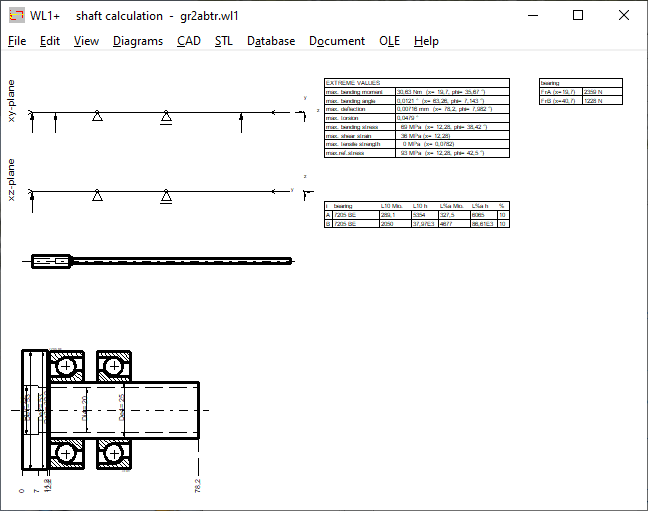GEO1 +: STL profile without numbers

The numbering of the coordinate points has been omitted for STL and CAD output, otherwise the numbers will appear in the CAD drawing or as 7-segment numbers in the 3D model.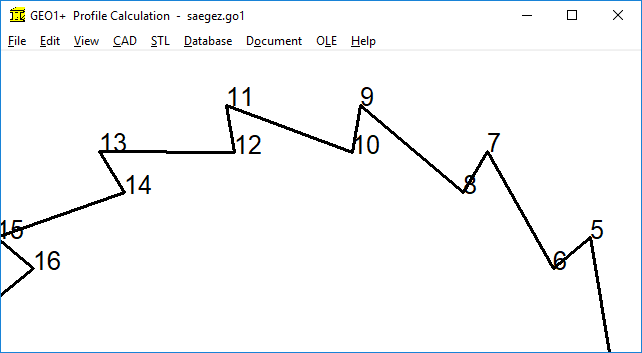Payment by Paypal instead of Mastercard / VISA

Payment with Mastercard or VISA card is no longer possible since December 1st, 2019. There is now payment via PayPal. You can create a Paypal account and configure your Mastercard or VISA to be charged.

Fixed network telephone connection problems

After our landline connections at Deutsche Telekom were terminated a long time ago due to unrecoverable malfunctions and converted to cable BW, the new fiber optic cable connection ran smoothly for a long time. However, the disorders have recently been increasing. Kabel-BW was taken over by Unitymedia and now Unitymedia was taken over by Vodafone. Maybe waiting for the 5G mobile network helps, then you no longer need a landline. In the event of a landline failure, please send an email. In the event of a fixed network failure, our Internet runs over the mobile network (with "Huawei Mobile WiFi").

EBIKE software: calculate the range of e-bikes and other vehicles

You can use the EBIKE mini program to calculate the range of your e-bike.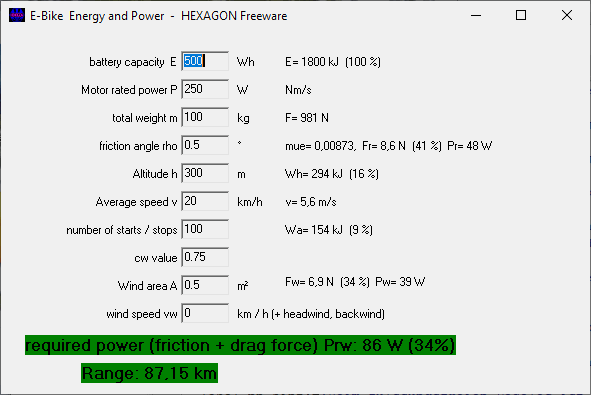Position energy, kinetic energy, friction work and air resistance are the main types of energy in vehicles.

Position energy if you overcome h meters of altitude uphill: E = m * g * h

Kinetic energy at start / stop with acceleration to speed v: E = ½ m * v²

Friction work with coefficient of friction µ and path s: E = m * g * µ * s

Air resistance at target area A and speed v: E = ½ * rhoair * cw * A * v² * s

With pedelecs and electric bicycles, the range was usually given in km, today only the capacity of the battery. Mostly in watt hours [Wh]: 1 Wh = 3600 Nm / s * s = 3600 Nm = 3600 J.

To calculate how far the battery charge lasts for a bike tour, the most important thing is the weight of the rider with a bike and the altitude difference.

The weight force of a rider 75 kg plus e-bike 25 kg results in a weight force FG = m * g = 1000 N. With 1 Wh = 3600 Nm you can lift a weight of 100 kg by 3.6 m. With a battery capacity of 500Wh, there are 1800 vertical meters. This could be used to cross the Alps if friction, air resistance and acceleration energy are not taken into account. The pedelec's own contribution by pedaling can be added to the battery capacity.

The work of friction is W = FG * µ * s with µ = coefficient of friction and s = distance traveled. The coefficient of friction can be determined on the inclined plane, from which angle the bike no longer rolls downhill. µ = sin alpha, at 1 ° (or 1.75%) slope µ = 0.0175

The range on a straight line is then s = E / (FG * µ). Then you come with 500 Wh and 100 kg on a straight line s = 500 * 3600 / (1000 * 0.0175) = 102857m, about 100 km away.

The acceleration work is also negligible if you rarely have to brake. To accelerate 100 kg to 20 kmh you need W = 0.5 * m * v² = 0.5 * 100 kg * (20 / 3.6) ² = 1543 Nm That is almost half a watt-hour.

The work to overcome air resistance is

W = rho / 2 * cw * A * v² * s

With air density 1.2 kg / m³, cw = 0.75 for seated people on bicycles and surface 150 cm * 40cm = 0.6m², W = 0.54 kg / m * v² * s

s = E / (0.54 * v²)

At 20 km / h = 5.55 m / s you need 500 Wh for 108 km for the air resistance. In the event of a headwind, the speed v must be increased by the wind speed.

If there are still vertical meters to be mastered, the route is calculated with

s = (E - FG * h) / (FG * µ) or s = (E / FG - h) / µ

Range [km] = E [Wh] * 360 / weight [kg] - vertical meters / (µ * 1000)

If you take a normal bike instead of an e-bike and do not want to lose weight, you have to add around 1800 kJ (430 kcal) for a battery charge of 500 Wh, as 150 grams of muesli or a bar of chocolate is enough. Around 1000 kilocalories for 1 kilowatt hour.

The range of other vehicles can also be calculated in this way. In the electric car, air resistance and electrical consumers (especially heating) have to be subtracted and, if necessary, the recuperation energy added when braking. In the electric car, a battery capacity of 0.5 kWh does not go far, here 20 to 100 kWh are the norm.

In order to calculate cars with combustion engines, the energy content must be converted into kWh. The efficiency up to the gearbox output must be taken into account.

Petrol: 2.8 kW / l (9.3 kWh / l * internal combustion engine efficiency 30%)

Diesel: 4 kWh / l (10 kWh / l * combustion engine efficiency 40%)

With a tank capacity of 50 l petrol, you then have approx. 140 kWh and with 50 l diesel even 200 kWh of energy in the tank.

In addition to the range, you can also calculate the maximum speed based on performance, wind attack area and drag coefficient. To do this, increase speed v until the calculated power for friction and air resistance is the same as the engine power.

And for an S-Pedelec you can calculate that an engine power of 250 W is not sufficient for a speed of 45 km/h on a level track.

Can you replace a battery with an electric motor as a vehicle drive with a flywheel with gearbox?

How much energy can be stored in a flywheel? It depends on the speed and moment of inertia. The most effective is a circular flywheel with a large outer diameter and thin wall.

Energy E = ½ J * omega²

Angular frequency omega = 2 pi n

Mass moment of inertia circular ring J = m * (r1² + r2²) / 2

Example Flywheel as e-bike drive between rim and rear wheel hub made of 10 mm thick steel: re = 250mm, ri = 240mm, 50 mm wide

m = 6 kg, J = 0.36 kgm²

E = J / 2 * omega² = 1776 kJ at 30,000 rpm

E = 1776 kJ at 30,000 rpm, which corresponds to almost 500 Wh and thus the capacity of an e-bike battery. Installed in the rear wheel hub of a bike, you would need an automatic transmission and a clutch for the wheel drive at the desired speed. The speed of a 28" wheel is only 120 / min at 20 km/h, then you need a gearbox with a gear ratio between 150 and 800. If the speed drops to half (15,000 / min), the flywheel accumulator is 75% discharged and should to be charged.

For practical use in an e-bike, one would have to take into account the effects of maintaining mass impulse when changing the direction of travel, whether unusual forces and moments can be compensated by the driver without any problems, or whether such a flywheel bike would only be suitable for a bicycle highway with a straight line.

But what speeds and forces occur with a flywheel with a diameter of 500 mm and 30,000 revolutions per minute?

Angular frequency omega = 2 * pi * n = 3141 / s

Peripheral speed at 30,000 / min: omega * r = 785 m/s (2800 km/h)

This is higher than the speed of sound, the flywheel must run in a vacuum.

Centrifugal force F = m * omega² * r = 14,800 kN

Tension: Sigma = F / A = 14800E3N / (50mm * 10mm) = 30000 N/mm²! (Safety 0.01)

Fperm = Sigma * A = 350N / mm² * 500mm² = 175 kN

Omega perm = sqrt (F / (m * r)) = 340 / s

n perm = 54 / s = 3260 / min

The 50 cm steel tire cannot withstand more than 3260 / min.

The energy at 3260 / min is only 173 kJ (48 Wh), which is clearly too little.

Because a flywheel storage can be charged quickly and can release its energy just as quickly, it is at most suitable as an additional booster, charged by recuperation when braking. But rather in a train, not in an e-bike.